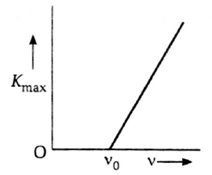The maximum kinetic energy Kmaxof photo-electrons emitted in a photoelectric cell is measured using lights of various frequencies v,. The slope of the graph is equal to

# The maximum kinetic energy $\left({\mathrm{K}}_{max}\right)$of photo-electrons emitted in a photoelectric cell is measured using lights of various frequencies v,. The slope of the graph is equal to1. A

charge on an electron

2. B

work-function of emitter

3. C

velocity of photon

4. D

Planck's constant

Register to Get Free Mock Test and Study Material

+91

Verify OTP Code (required)